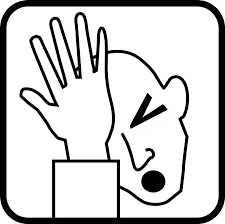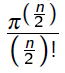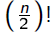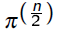BrainDen.com - Brain Teasers

## Question

This puzzle is inspired by the seventh of Rocdocmac's Difficult Sequences:

In n dimensions, where a point is an n-tuple of coordinate values { x1x2,  x3,  ... , ,xn },
the unit sphere is the locus of points for which

x12+ x22 + x32 + ... + xn2 = 1.

In one dimension this is a pair of points that encloses a volume (length) of 2 and comprises a surface area of 0. In two dimensions it's a circle that encloses a volume (area) of pi and comprises a surface area (circumference) of 2 pi.

So the enclosed volume and surface area both start out, at least, as increasing functions of n.

What happens as n continues to increase?

Puzzle: Is there a value of n for which the volume reaches a maximum?

Bonus question: What about the surface area?

## Recommended Posts

• 0
Spoiler

Yes there is a maximum!

If the volume of only the unit ball/sphere (r=1) is considered, the maximum occurs in dimension 5. Thereafter, the volumes decrease and tend to zero as the dimension goes to infinity.

However, as the radius of the sphere increases, the maximum does not necessarily occur in dimension 5. The maximum would then be reached in higher dimensions as the radius increases.

I believe that surface area follows a similar pattern, but have not tested it yet.

Here are the unit volumes for the first 10 dimensions [dimension - volume (r=1)] ...

1 - 2

2 - 3.1416

3 - 4.1888

4 - 4.9348

5 - 5.2638

6 - 5.1677

7 - 4.7248

8 - 4.0587

9 - 3.2985

10 - 2.5502

##### Share on other sites
• 0
Spoiler

Surface area has a maximum in dimension 7 if r=1 (unit), then decreases and tends to zero.

##### Share on other sites
• 0
Spoiler

Graphs for increase in n for volume and surface area of spheres. Unit volumes/areas (r = 1) at the top and other radii at the bottom. Interactive sheet in which the radius can be changed by typing in a value for r in the green box. Maxima for r = 1 exist for volume (5D) and area (7D)

Calculated.xlsx

##### Share on other sites
• 0

That's very cool. It got me thinking.

The sphere encloses the greatest volume for a given surface area.
I don't know if this makes sense to ask but I'll try, anyway.

For what dimension of space is the volume to surface ratio of a unit sphere the greatest?
For example, in 3-D it's r/3 = 1/3.

##### Share on other sites
• 0
Spoiler

Maximum V/A ratio (r=1) ... if not D1 (=infinity), then D2 (=0.5). Moreover, the greatest difference (A-V) is for D8 (~28.411), whereas maximum for A+V it is D7 (~37.798). And max A^V? D8

Edited by rocdocmac

##### Share on other sites
• 0
Spoiler

Oops!

Maximum V/A ratio = 0.5 (D2). It's zero for D1, not infinity!

Spoiler##### Share on other sites
• 0

Question: the process shown demonstrates a local maximum for the function, but is there a way to prove that (for instance) at n=1000 it doesn't suddenly reach a new maximum? (I guess what I'm trying to say is, is there a proof, or is the solution presented here just process of elimination?)

##### Share on other sites
• 0
Spoiler

The general formula for the volume of an even-dimensional n-sphere is ...After the maximum unit volume in D7 is reached, the ratio starts dropping sincegrows much faster than.

So by the time you reach n=1000 (or higher towards infinity), the value for the numerator cannot compete with that of the denominator at all and therefore a second maximum cannot occur.

##### Share on other sites
• 0
19 hours ago, rocdocmac said:
Hide contents

The general formula for the volume of an even-dimensional n-sphere is ...

After the maximum unit volume in D7 is reached, the ratio starts dropping sincegrows much faster than.

So by the time you reach n=1000 (or higher towards infinity), the value for the numerator cannot compete with that of the denominator at all and therefore a second maximum cannot occur.

Nice, thanks!

## Join the conversation

You can post now and register later. If you have an account, sign in now to post with your account.×   Pasted as rich text.   Paste as plain text instead

Only 75 emoji are allowed.

×   Your previous content has been restored.   Clear editor

×   You cannot paste images directly. Upload or insert images from URL.

×